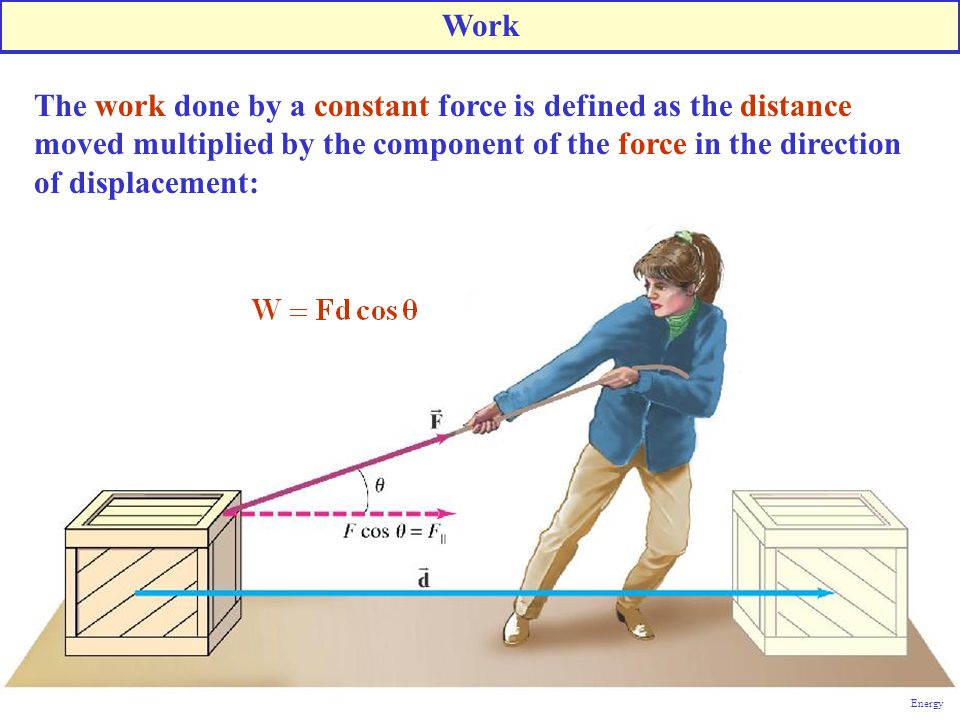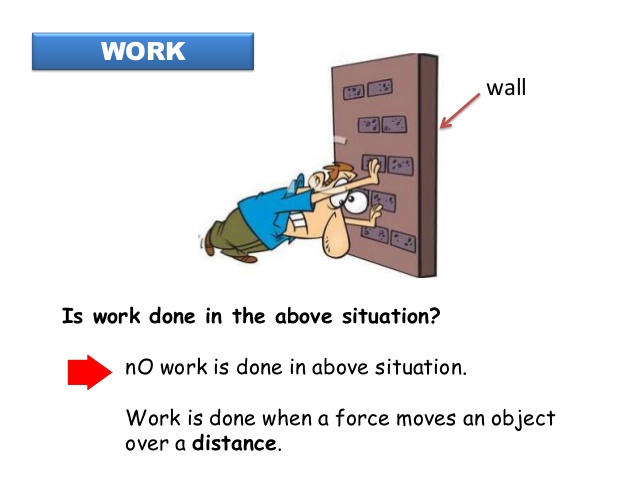# Calculation Involving Work Done

Welcome to Class !!

In today’s Basic Science class, We will be discussing Calculation Involving Work done. We hope you enjoy the class!

## TOPIC:  CALCULATION INVOLVING WORK DONE

On the basis of the direction of motion of a body and the direction of force applied, the way of calculation of work done is determined. Try this simple activity to help you better understand this concept.

Release a metal ball A so that it rolls down a slope and hits another ball B at the bottom of the slope. What happens? Repeat this with balls of several sizes placed at the same point at the bottom of the slope. If the ball B on the ground is much heavier than the one that hits it, it does not move very far. When the ball B moved, ball A must have exerted a push on B to make it move. The rolling ball A gives some energy to the ball B that it collides with; we say that it has done work on ball B. The product of the push and the distance through which ball B moves in the direction of the push is defined as the work done. Thus:

Work = force x distance moved in the direction of the forceThis work is a measure of the energy transferred to the ball B by ball A that collided with it. The unit of work is, therefore, the unit of force x the unit of distance. We already know the unit of the force to be newton (N) while that of distance is the metre (m). So, the unit of work is the newton metre (Nm), which is called the joule, (J), after J.P. Joule, a scientist who did a great deal of experimenting with energy.

If a force of 10N moves through a distance of 1m in the direction of the force, the work done = 10 x 1 = 10J. Similarly, if a force of 20N moves through 0.5m, the work done = 20 x 0.5 = 10J. Here are some examples below:

1. What work is done when a mass of 25.00kg is raised through a vertical height of 4.5m (acceleration due to gravity is 10m/sec)?

Solution:

Work done = force x distance

Work done = mass × acceleration × distance

Since force = mass × acceleration

Where mass = 25kg; acceleration = 10m/s; distance = 4.5m

Therefore work done = 25.00kg × 10m/s × 4.5m = 1125 joules1. Calculate the work done by a body of weight 100N which, moves through a distance of 15N

Solution:

Force = 100N

Distance moved = 15m

Work done = force × distance moved

= (100 x 15) joules

= 1500 joules.

1. Find the work done by a man who lifts a brick of 50N from the ground to a vertical height of 6m by means of a rope.

Solution:

W = ? F = 50N, S = 6m

Work done = Force × distance

W = 50 × 6 = 300 Joules

You can try out other calculations on your own now.

We have come to the end of this class. We do hope you enjoyed the class?

Should you have any further question, feel free to ask in the comment section below and trust us to respond as soon as possible.

In our next class, we will be talking about Energy Transfer when Work is Done. We are very much eager to meet you there.

Are you a Parent? Share your quick opinion and win free 2-month Premium Subscription

Don`t copy text!